Processing & Handling

# Compressible Fluid Flow Calculation Methods

| By Fucheng Teng, Pedro Medina and Mark Heigold, Jacobs Canada, Inc.

Compressible gas flow with significant variation in density along pipes is commonplace in the chemical processing industries (CPI). In designing these pipes for compressible flow, it is important to calculate the pressure loss or maximum gas-handling capacity for safety and economic reasons.

Due to the complexity of the equations for compressible flow, which often require time-consuming iterations, current engineering practice considers three special flow conditions to simplify calculations: incompressible (fluid density is constant), isothermal (fluid temperature is constant) and adiabatic (there is no heat transfer between the fluid and its surroundings). However, when flow conditions are unknown, and assumptions must be made, engineers can become concerned with the accuracy of calculations. Through thorough derivations and analysis of literature, this article will delve deeper into pressure-drop calculation methods to form a valid comparison of each method.

Amidst the confusion of choosing the proper pressure-drop calculation method, two questions arise that must be addressed. Firstly, engineers may wonder which equation is more conservative, in terms of pressure-drop calculations: isothermal or adiabatic? API Standard 521  recommends the isothermal method to size all pipes in relief systems, with the exception of cryogenic conditions, where the adiabatic equation is preferable. Yu  finds that the isothermal equation method is not always as conservative when compared with the adiabatic method, which is sometimes more conservative depending on inlet pressure and other fluid properties.

The second major concern engineers may have is determining when it is appropriate to assume incompressible flow. Accepted literature  concludes that the incompressible equation can be applied if the density does not vary by more than around 30%. It is reported  that the incompressible equation can be employed using an average density when the pressure drop is less than 40% of the inlet pressure. This article will clarify these concerns and will demonstrate which pressure-drop calculation methods are most appropriate in various scenarios.

## Compressible fluid flow

First, the basics of fluid flow in pipes must be discussed. The flow of an ideal gas through a horizontal pipe with constant cross-sectional area is governed by Newton’s second law, the first law of thermodynamics, the ideal gas law and the law of conservation of mass. Equation (1) defines Newton’s second law. For definitions of the symbols and abbreviations used throughout this article, please refer to the “Nomenclature” section.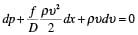( 1)

The first law of thermodynamics (steady-state) is shown in Equation (2), where h is specific enthalpy.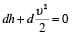(2)

The ideal gas law, shown in Equation (3), is crucial for fluid-flow calculations.

pv = nRT (3)

Equation (4) illustrates the law of conservation of mass.

dpv = 0 (4)

The conditions corresponding to incompressible, isothermal and adiabatic flow must also be defined. These are shown in Equations (5), (6) and (7) below.

Incompressible flow:

= 0 (5)

Isothermal flow:

dT = 0 (6)

dh = –cpdT (7)

By inserting any of Equations (5), (6) and (7) into Equations (1) through (4), the incompressible, isothermal and adiabatic flow equations can be derived accordingly.

Another important term that must be defined is the Mach Number (Ma), which is the ratio of gas velocity to the local sonic velocity, as shown in Equation (8).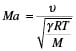(8)

When Ma ≥ 1 (gas velocity exceeds sonic velocity), sonic choking occurs. The expression for Mach number is independent of flow conditions.

## Isothermal flow

In isothermal flow, the fluid temperature remains constant. By using Equations (1) through (4) and Equation (6), Equations (9) and (10) for isothermal flow of ideal gases at known upstream or downstream conditions can be derived .

Equation (9) is used when upstream conditions are known. Here, Mi is used to denote Mach Number, as the specific heat ratio γ is not present.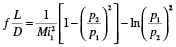(9)

Equation (10) is used when downstream conditions are known.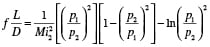(10)

In Equations (9) and (10), Mi1 and Mi2 are inlet Mach number and outlet Mach number, respectively, and they are given by Equations (11) and (12).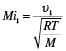(11)(12)

Unlike Equation (8), Equations (11) and (12) do not have a physical meaning, they are just the result of grouping υ2, T and M together when deriving the isothermal equation . In order to distinguish from the Mach number expressed in Equation (8), Mi is used instead of Ma when there is no specific heat ratio (γ) in the expressions throughout this article. It can be simply demonstrated that the maximum Mi1 is equal to one based on two boundary conditions: fL/D ≥ 0 and 0 < p2/p1 ≤ 1. Since the ratio of Mi to Ma is equal to the square root of γ the isothermal flow will be choked when Ma equals one over the square root of γ.

An isothermal flow chart developed by Mak  is one of two graphical methods that have been adopted by API Standard 521  to size discharge pipes for relief devices. In the chart, p2/p1 is plotted against fL/D using either Equation (9) with known inlet conditions or Equation (10) with known outlet conditions. The interested reader is referred to Branan’s book  for the detailed procedure on how to use Mak’s chart. In this article, Mak’s chart is extensively used to compare the aforementioned three pressure-drop calculation methods.

Adiabatic flow has no heat transfer into or out of the fluid. Adiabatic conditions prevail if the pipe is well insulated or if the heat transfer rate is very small compared to the fluid flow. The adiabatic flow expression illustrated in Equation (13) can be derived by using Equations (1) through (4) and Equation (7).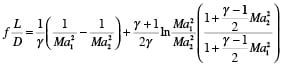(13)

There are both inlet and outlet Mach numbers in Equation (13), in contrast to only one Mach number in the isothermal equation expressions derived in Equations (9) and (10). In order to plot the adiabatic flow equation in Mak’s chart, we have to find the relationship between Ma1 and Ma2 and eliminate one of them from Equation (13). The ratio of downstream to upstream pressure is given by Equation (14).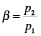(14)

The ratio of squared upstream to downstream Mach number is denoted below in Equation (15).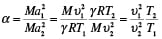(15)

Using the ideal gas law, we arrive at Equation (16).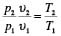(16)

Rearranging Equations (14), (15) and (16) gives expressions for υ1 and T1, shown respectively in Equations (17) and (18).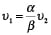(17)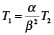(18)

For an ideal gas, we know that dh = cpdT and cp = γR/(γ – 1). Integrating Equation (2) from inlet to outlet yields Equation (19).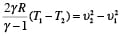(19)

Assuming that T2 > T1 in Equation (19), T1 and υ1 can be eliminated by inserting Equations (17) and (18) into Equation (19), resulting in Equation (20).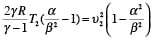(20)

Rearranging Equation (20) gives Equation (21).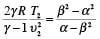(21)

By grouping T2 and υ2, Equation (21) becomes Equation (22).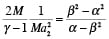(22)

Equation (23) simplifies matters by denoting the lefthand side of Equation (22) as φ.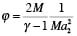(23)

Solving for the roots of Equation (22), and knowing that both α and φ are greater than zero, the expression’s valid root is given in Equation (24).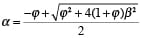(24)

By inserting Equation (15) into Equation (13), we get Equation (25).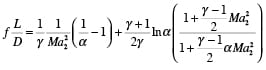(25)

The term α is expressed as in Equation (24), or, alternatively, as shown as in Equations (26) and (27).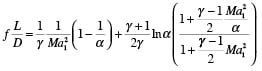(26)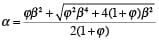(27)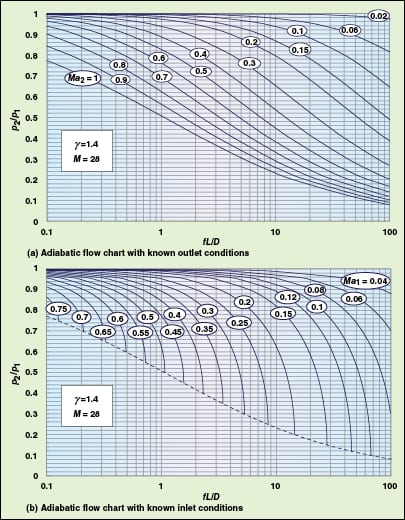Figure 1. These typical adiabatic flow charts can be used to determine pressure drop for known inlet or outlet piping conditions

With only one Mach number in Equations (25) and (26), we can now plot the adiabatic flow equation using Mak’s chart. A typical graphical representation of Equations (25) and Equation (26) is shown in Figure 1, for known outlet and inlet conditions. In Figure 1b, the dashed line represents the boundary between the subsonic and supersonic regions. Ma2 is equal to one along the dashed line.

From Equations (25) and (26), we can also see that p2/p1 is affected by pipe data (fL/D), Mach number (Ma1 or Ma2), specific heat ratio (γ) and molecular weight (M). Figure 1 clearly shows how p2/p1 varies with different fL/D and Mach number. In the following section, the effect of molecular weight and specific heat ratio will be investigated.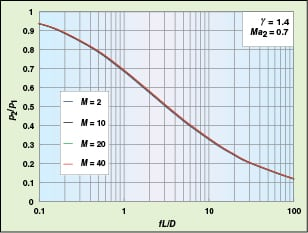Figure 2. The effect of molecular weight on adiabatic pressure drop with known outlet conditions is minimal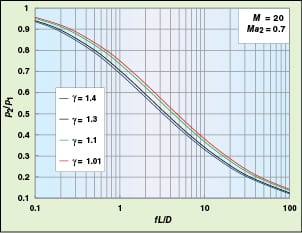Figure 3. Specific heat ratio’s effect on pressure drop is shown for fluid in adiabatic flow with known outlet conditions

Four virtual fluids with the same specific heat ratio (γ = 1.4) and different molecular weight (2, 10, 20 and 40) are selected for comparison. They pass through the same pipe (diameter and length) at the same mass flowrate. The outlet pressure (p2) and Mach number (Ma2) for all four fluids are identical. The calculated p2/p1 change with fL/D is shown in Figure 2. The clustering of the four curves in Figure 2 indicates that the effect of molecular weight on pressure drop is negligible.

Similarly, the p2/p1 values of four fluids with the same molecular weight and different γ is plotted against fL/D. In contrast to Figure 2, Figure 3 shows four curves with wide separation, indicating that, unlike molecular weight, specific heat ratio significantly affects pressure drop. At the same pipe length, p2/p1 decreases with increasing specific heat ratio. Since all four fluids have the same outlet pressure (p 2), a smaller p2/p1 in Figure 3 corresponds to larger p1 and larger pressure drop. At fL/D values of around one, the calculated p1 for a fluid with γ of 1.4 is about 6% higher than that for fluid with γ of 1.1.

With the adiabatic flow equation plotted in Mak’s chart, we now can compare isothermal and adiabatic flow in graphical form. A virtual fluid with γ = 1.4 and M = 20 is chosen for the comparison. The fluid passes through a pipe with a length of L and an internal diameter of D. The real flow condition is unknown, instead, we assume isothermal and adiabatic conditions for the pressure-drop calculation. The results are then compared. Two scenarios are considered in the calculation, one with known inlet conditions, and one with known outlet conditions. For both isothermal and adiabatic flow, the Mach number (Ma) based on sonic velocity, as defined in Equation (8), is used in the comparison.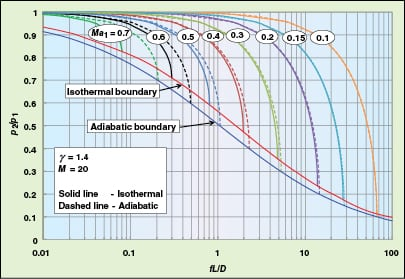Figure 4. With known inlet conditions, adiabatic and isothermal fluid flow equations are compared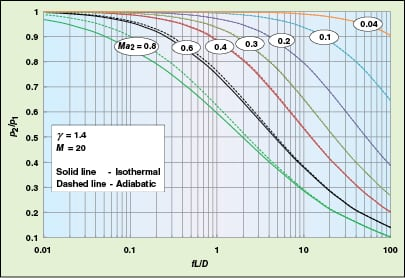Figure 5. With known outlet conditions, adiabatic and isothermal fluid flow equations are compared

Scenario 1: known inlet conditions. With inlet conditions p1, υ1, T1 and Ma1 known, outlet conditions p2, υ2, T2 (which is the same as T1 for isothermal flow) and Ma 2 must be calculated. Using Equations (9) and (26), adiabatic and isothermal curves are plotted for p2/p1 versus fL/D in Figure 4, where the red and blue lines represent the boundary between subsonic and supersonic region. In Figure 4, it is notable that, at large Ma1 values, the solid line is below the dashed line, which means that calculated p2 values for isothermal flow are smaller than that for adiabatic flow at the same fL/D.

In other words, isothermal flow provides more conservative results regarding pressure-drop calculations. Also, it should be noted that the solid and dashed lines nearly overlap with each other at small values of Ma1, implying that the difference between isothermal and adiabatic flow assumptions is insignificant. The discrepancy only becomes obvious when the Mach number is very large (>0.3). Additionally, the red line is above the blue line, which shows that the critical pressure at the pipe’s outlet (p2), for a given flowrate and pipe length, is lower for adiabatic flow than for isothermal flow.

Scenario 2: known outlet conditions. In the next scenario, p1, υ1, T1 and Ma1 under isothermal and adiabatic flow are to be calculated and compared using known outlet parameters (p2, υ2, T2 and Ma2). The result is shown in Figure 5. Similar to the previous scenario with known inlet conditions, the solid line and dashed line almost overlap with each other at small Mach numbers (Ma2 < 0.3), implying that the difference between the two methods is negligible. When Ma2 becomes larger (Ma2 ≥  0.4), the two lines separate and the solid line is below the dashed line, suggesting that isothermal flow gives a larger p1 value than predicted by adiabatic flow. It is also worth mentioning that the difference between isothermal and adiabatic flow is small at large fL/D values (greater than 10) regardless of the Mach number. Thus, we can apply either isothermal or adiabatic flow equations in this scenario.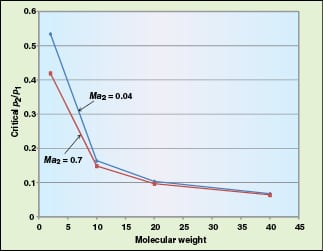Figure 6. As molecular weight decreases, so does critical pï»¿ï»¿ï»¿2ï»¿/pï»¿ï»¿ï»¿1ï»¿

Overall, the isothermal flow equation is more conservative than the adiabatic equation in terms of pressure-drop calculations, because the solid line is below the dashed line in Figures 4 and 5 at most conditions. However, the question arises as to whether isothermal flow is always conservative, even when the solid and dashed lines are visually inseparable. A careful look at Figures 4 and 5 finds that the answer is “yes” for known inlet conditions but “no” for known outlet conditions, in which the dashed line turns out to be above the solid line when p2/p1 decreases to a critical value. This critical p2/p1 value is found to be greatly dependent on the fluid’s molecular weight, but not on specific heat ratio. As shown in Figure 6, criticalp2/p1 continues to decrease with molecular weight. For hydrogen with a molecular weight of 2 and a specific heat ratio of 1.3, the adiabatic equation becomes more conservative (larger p1) as p2/p1 drops below 0.53 when the outlet Mach number Ma2 is 0.04.

## Temperature and velocity

The pressure drop and variation in temperature and velocity along a pipe are always interrelated. With known inlet conditions, the dimensionless temperature (T2/T1) and velocity profiles (υ21) for both isothermal and adiabatic flow are depicted in Figure 7. At both small and large Mach number values, it can be clearly seen that υ 21 under isothermal flow is larger than that under adiabatic flow at the same pipe length (fL/D). Since higher velocity will result in larger pressure drop, Figure 7 indirectly explains why isothermal flow is more conservative in term of pressure-drop calculations. Figure 7a also shows that the temperature drop for adiabatic flow is very small (< 0.1% when fL/D < 50).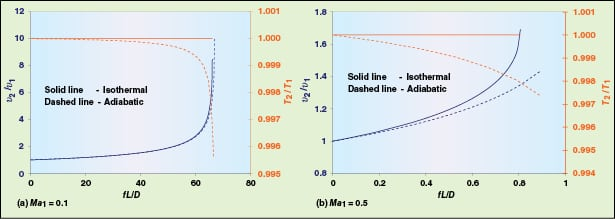Figure 7. Velocity and temperature profiles of isothermal and adiabatic flow with known inlet conditions for two different Maï»¿ï»¿ï»¿1ï»¿ values show that isothermal flow assumptions are generally more conservative in terms of pressure-drop calculations

So we may conclude that the temperature remains constant at a small inlet Mach number (Ma1), even if the flow is adiabatic. Comparing Figure 7a and Figure 4, we can understand why the solid and dashed lines overlap each other when Ma1 is less than 0.3.

## Critical mass flux

Critical mass flux under isothermal flow is defined as the maximum mass flowrate per unit duct area at Mi1 = 1. It is reported by Lapple  and referenced in API Standard 521  that the critical mass flux of adiabatic flow (where γ = 1.4) is 12.9% higher than that of isothermal flow under the same inlet conditions. However, Lappel’s model represents gas expansion from an infinite reservoir through a frictionless convergent nozzle, which has an inlet velocity equal to zero. This assumption is not valid for gas flowing in pipelines. With the equations derived in this article, we can calculate and compare the critical mass flux for isothermal and adiabatic flow and find that the reported value of 12.9% is not accurate. The detailed calculations are presented below. First, we define critical mass flux under isothermal flow at Mi1= 1 as the maximum mass flux (Gmax) in Equation (28).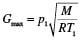(28)

At any pipe length, Gmax under isothermal flow can be expressed as Equation (29).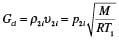(29)

Where p2i and υ2i are outlet pressure and velocity when the conditions in Equation (30) are true.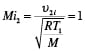(30)

Therefore, the ratio of critical mass flux at any pipe length to maximum mass flux under isothermal flow is given in Equation (31).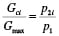(31)

By solving Equation (10) and setting Mi2 equal to one, p2/p1 can be determined. At any pipe length, the critical mass flux under adiabatic flow can be expressed as Equation (32).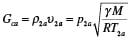(32)

The ratio of critical mass flux under adiabatic flow to maximum mass flux under isothermal flow is shown by Equation (33).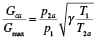(33)

Based on Equations (14) through (17), we arrive at Equation (34).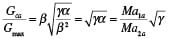(34)

At critical flow, Ma2a is equal to one, so Equation (34) is simplified to Equation (35).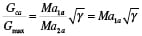(35)Figure 8. The ratio of critical mass flux to maximum mass flux as a function of pipe length varies with specific heat ratio

In Equation (35), Ma1a can be calculated by solving Equation (13) at any fL/D and setting Ma2a equal to one. Equations (31) and (35) are plotted in Figure 8 for three fluids with different specific heat ratios. At any pipe length, the ratio of critical mass flux of adiabatic flow to isothermal is given by Equation (36).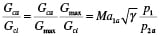(36)

Equation (36) can be simplified to Equation (37) since p1/p2a and Ma1a equal one when the square root of fL/D equals zero.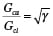(37)

At γ = 1.4, Gca/Gci is equal to 1.183, so the critical mass flux under adiabatic flow is 18.3% higher than that under isothermal flow, which is different from the reported value of 12.9% from API Standard 521.

## Isothermal versus incompressible

In deciding whether to apply the incompressible flow equation for compressible fluid flow conditions, engineers often rely on certain rules of thumb. However, some of these rules of thumb can be quite misleading . In the following section, we examine the difference between isothermal and incompressible equations for pressure-drop calculations.

For an ideal gas flowing through a horizontal pipe, Equation (38) shows that the total pressure drop is the summation of pressure drop caused by friction and acceleration.

âˆ†pTotal = âˆ†pFriction + âˆ†pAcceleration   (38)

For incompressible flow, the acceleration term is negligible. So Equation (38) becomes Equation (39).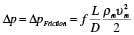(39)

In Equation (39), ρm and υm are the averaged density and velocity, which are defined in Equations (40) and (41), respectively.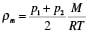(40)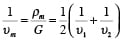( 41)

Moving fL/D to the lefthand side in Equation (39) gives Equation (42).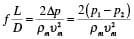(42)

Substituting ρm and υm with Equation (40) and (41) in Equation (42) results in the expression shown in Equation (43).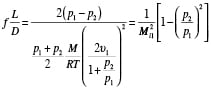(43)

Equation (43) can be transformed to Equation (44) with a known outlet Mach number Mi2.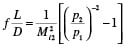(44)

Equations (43) and (44) are the incompressible flow equations. Comparing with the isothermal equation defined in Equation (9), it is seen that the natural log term is cancelled out. Since pressure drop due to acceleration is negligible for incompressible flow, it can be deduced that the natural log term in Equation (9) accounts for acceleration and the first term accounts for friction, as illustrated in Equation (45).(45)

The difference between the calculated pressure using the isothermal equations defined by Equations (9) and (10) and the incompressible equations defined by Equations (43) and (44) is shown in Tables 1A and 1B for both known inlet and outlet conditions. The difference is expressed for known outlet and inlet conditions by Equations (46) and (47), respectively.

For known outlet conditions: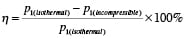( 46)

For known inlet conditions: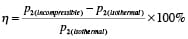(47)

It can be seen from Table 1 that η depends on both pressure drop (p2/p1) and Mach number (Mi). At certain Mi values, η is at its maximum at p2/p1 values of around 0.7–0.8 and gradually decreases with p2/p1. However, η might not be practically correct when p2/p1 is very small since it requires immense energy to maintain constant temperature. The values with a difference larger than 1% are highlighted in green in Table 1. Since Equations (43) and (44) do not contain natural log terms, we can very quickly size compressible fluid pipe with known error as shown in Table 1. Detailed below are the steps for calculating p2 using Equations (43) and (44). By rearranging Equation (44), we arrive at Equation (48), an expression for p2/p1.

 Table 1A. Mach Number at pipe outlet (Mi2) p2/p1(incompressible) 0.01 0.05 0.1 0.2 0.3 0.4 0.5 0.6 0.7 0.8 0.9 1 0.01 0.00% 0.00% 0.00% 0.00% 0.00% 0.01% 0.01% 0.02% 0.02% 0.03% 0.04% 0.05% 0.05 0.00% 0.00% 0.01% 0.03% 0.07% 0.12% 0.19% 0.27% 0.37% 0.48% 0.60% 0.74% 0.1 0.00% 0.01% 0.02% 0.09% 0.21% 0.37% 0.57% 0.82% 1.11% 1.45% 1.83% 2.25% 0.2 0.00% 0.02% 0.06% 0.26% 0.58% 1.02% 1.59% 2.27% 3.07% 3.97% 4.98% 6.08% 0.3 0.00% 0.03% 0.11% 0.43% 0.97% 1.71% 2.66% 3.80% 5.12% 6.61% 8.26% 10.04% 0.4 0.00% 0.04% 0.15% 0.59% 1.31% 2.32% 3.61% 5.15% 6.95% 8.97% 11.21% 13.61% 0.5 0.00% 0.04% 0.17% 0.69% 1.56% 2.77% 4.31% 6.19% 8.38% 10.86% 13.61% 16.59% 0.6 0.00% 0.05% 0.18% 0.74% 1.67% 2.98% 4.68% 6.77% 9.26% 12.12% 15.33% 18.84% 0.7 0.00% 0.04% 0.18% 0.71% 1.60% 2.90% 4.61% 6.77% 9.42% 12.56% 16.18% 20.21% 0.8 0.00% 0.04% 0.14% 0.58% 1.34% 2.45% 3.97% 5.98% 8.56% 11.81% 15.76% 20.38% 0.9 0.00% 0.02% 0.09% 0.35% 0.82% 1.53% 2.56% 4.01% 6.07% 8.96% 12.99% 18.30% 0.91 0.00% 0.02% 0.08% 0.32% 0.75% 1.41% 2.36% 3.72% 5.68% 8.48% 12.47% 17.85% 0.92 0.00% 0.02% 0.07% 0.29% 0.68% 1.28% 2.15% 3.42% 5.25% 7.94% 11.88% 17.34% 0.93 0.00% 0.02% 0.06% 0.26% 0.61% 1.14% 1.93% 3.09% 4.79% 7.34% 11.20% 16.73% 0.94 0.00% 0.01% 0.06% 0.23% 0.53% 1.00% 1.70% 2.73% 4.28% 6.67% 10.41% 16.02% 0.95 0.00% 0.01% 0.05% 0.19% 0.45% 0.85% 1.46% 2.35% 3.73% 5.91% 9.50% 15.16% 0.96 0.00% 0.01% 0.04% 0.16% 0.37% 0.70% 1.20% 1.95% 3.12% 5.06% 8.41% 14.12% 0.97 0.00% 0.01% 0.03% 0.12% 0.28% 0.53% 0.92% 1.51% 2.46% 4.08% 7.10% 12.79% 0.98 0.00% 0.00% 0.02% 0.08% 0.19% 0.36% 0.63% 1.05% 1.72% 2.94% 5.45% 11.03% 0.99 0.00% 0.00% 0.01% 0.04% 0.10% 0.19% 0.32% 0.54% 0.91% 1.61% 3.27% 8.37% 0.992 0.00% 0.00% 0.01% 0.03% 0.08% 0.15% 0.26% 0.44% 0.73% 1.31% 2.73% 7.63% 0.995 0.00% 0.00% 0.01% 0.02% 0.05% 0.09% 0.16% 0.28% 0.47% 0.84% 1.84% 6.23% 0.999 0.00% 0.00% 0.00% 0.00% 0.01% 0.02% 0.03% 0.06% 0.10% 0.18% 0.41% 2.99%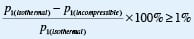(a) Calculated inlet pressure difference (at known outlet conditions) between using the incompressible and isothermal equations (η >1% is highlighted green)

 Table 1b. Mach Number at pipe inlet (Mi1) p2/p1(incompressible) 0.01 0.05 0.1 0.2 0.3 0.4 0.5 0.6 0.7 0.8 0.9 0.01 0.05 15.56% 0.1 2.41% 0.2 0.41% 12.97% 0.3 0.13% 3.63% 20.26% 0.4 0.06% 1.49% 6.77% 0.5 0.03% 0.71% 3.02% 17.26% 0.6 0.01% 0.36% 1.49% 7.14% 26.14% 0.7 0.01% 0.18% 0.75% 3.34% 9.36% 29.04% 0.8 0.00% 0.09% 0.36% 1.52% 3.89% 8.66% 22.34% 0.9 0.00% 0.03% 0.13% 0.55% 1.35% 2.72% 5.19% 10.71% 0.91 0.00% 0.03% 0.12% 0.48% 1.17% 2.35% 4.44% 8.81% 0.92 0.00% 0.02% 0.10% 0.42% 1.01% 2.01% 3.75% 7.23% 21.16% 0.93 0.00% 0.02% 0.08% 0.35% 0.85% 1.70% 3.13% 5.88% 14.05% 0.94 0.00% 0.02% 0.07% 0.29% 0.71% 1.40% 2.56% 4.71% 10.16% 0.95 0.00% 0.01% 0.06% 0.24% 0.57% 1.13% 2.04% 3.68% 7.43% 0.96 0.00% 0.01% 0.04% 0.19% 0.44% 0.87% 1.56% 2.78% 5.33% 0.97 0.00% 0.01% 0.03% 0.14% 0.32% 0.63% 1.13% 1.97% 3.63% 9.12% 0.98 0.00% 0.01% 0.02% 0.09% 0.21% 0.41% 0.72% 1.24% 2.23% 4.78% 0.99 0.00% 0.00% 0.01% 0.04% 0.10% 0.20% 0.35% 0.59% 1.03% 2.03% 0.992 0.00% 0.00% 0.01% 0.03% 0.08% 0.16% 0.27% 0.47% 0.81% 1.58% 5.37% 0.995 0.00% 0.00% 0.01% 0.02% 0.05% 0.10% 0.17% 0.29% 0.50% 0.95% 2.66% 0.999 0.00% 0.00% 0.00% 0.00% 0.01% 0.02% 0.03% 0.06% 0.10% 0.18% 0.44%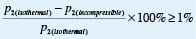(b) Calculated outlet pressure difference (at known inlet conditions) between using the incompressible and isothermal equations (η >1% is highlighted green)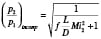( 48)

For given pipe characteristics (length, diameter and roughness), fluid flowrate, Mi2 and p2, fL/D is first calculated — f can be read from a Moody diagram or calculated with various equations. Next, we must calculate p2/p1 using Equation (48). Afterwards, we determine η from Table 1A based on Mi2 and p2/p1(incomp.). If η is acceptable, then p1 is reported as the final result. If η is not acceptable, the isothermal expression in Equation (10) must be used to re-calculate p2/p1(isothermal) and report a new p1 value.

## Modified incompressible flow

From Table 1 and Equations (43) and (44), it can be seen that the isothermal flow equation is more conservative than the incompressible equation because the latter does not include the acceleration term. Here, we present a modified incompressible equation to account for the missing acceleration term in Equations (43) and (44). Equation (49) defines fa, the acceleration factor, where υm and pm are averaged velocity and pressure.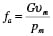(49)

The total pressure drop and pressure drop due to friction can be linked as in Equation (50) below .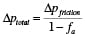(50)

Further expanding Equation (49) gives Equations (51) and (52).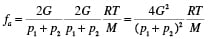(51)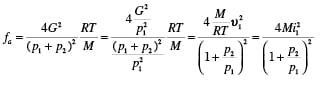(52)

The pressure drop due to friction is expressed in Equation (39). By substituting υm and pm, Equation (39) becomes Equation (53).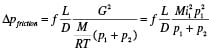(53)

Inserting Equations (51) and (53) into Equation (50) yields Equation (54).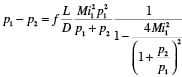(54)

Moving fL/D to the lefthand side brings us to the expressions in Equations (55) and (56).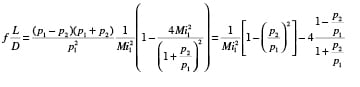(55)

With known outlet conditions, Equations (55) becomes Equation (56).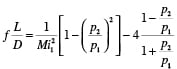(56)

Equation (56) is the modified incompressible flow equation. Comparing Equation (56) with Equation (43), it can be seen that the second term on the righthand side accounts for acceleration. Equation (56) and Equation (10) are plotted in Figure 9, which shows the solid and dashed lines overlapping each other at all chosen values for the Mach number. Therefore, the modified incompressible flow equation can be used to size pipes where fluid flow is compressible.

## Conclusions

1. For pipe sizing with compressible fluids, the isothermal flow equation is preferable since it gives a more conservative pressure drop estimate in the scope of practical engineering design.

2. When considering whether it is acceptable to use the incompressible equation to size gas pipes, both pressure drop and Mach number should be considered.

3. For a given pipe length and diameter, the critical mass flux under adiabatic conditions is larger than that under isothermal conditions. The maximum ratio between critical mass flux under adiabatic and isothermal condition is equal to the square root of γ.

4. Under adiabatic flow, specific heat ratio has significant effect on pressure drop, but the effect of molecular weight is negligible.

With these conclusions in mind and the equations derived in this article, engineers can begin to make educated assumptions when they are asked to size and determine pressure drop for pipes with compressible fluid flow.

Edited by Mary Page Bailey

## References

1. American Petroleum Institute, Standard 521, Pressure-relieving and Depressuring Systems, 5th Ed., Washington D.C., 2007.

2. Yu, F.C., Compressible Fluid Pressure Drop Calculation-Isothermal Versus Adiabatic, Hydrocarbon Processing, pp. 89–95, May 1999.

3. Darby, Ron, “Chemical Engineering Fluid Mechanics”, 2nd Ed., CRC, 2001.

4. Flow of Fluids Through Valves, Fittings, and Pipe, Technical Paper No.410, Crane Co., Joliet, Ill., 1988.

5. Saad, M.A., “Compressible Fluid Flow”, 2nd Ed., Prentice-Hall, Englewood Cliffs, NJ, 1998.

6. Mak, H.Y., New Method Speeds Pressure-Relief Manifold Design, Oil and Gas Journal, Nov. 1978.

7. Branan, Carl R., “Rules of Thumb for Chemical Engineers”, 4th Ed., Gulf Professional Publishing, Burlington, Mass., 2005.

8. Lapple, C.F., “Fluid and Particle Mechanics”, University of Delaware, Newark, Del., 1951.

9. Walters, T., Gas-Flow Calculations: Don’t Choke, Chem. Eng., Jan. 2000.

## AuthorsFucheng Teng is a process technologist/specialist at Jacobs Canada Inc. (205 Quarry Park Blvd. S.E., Calgary, Alberta, Canada T2C3E7; Phone: +1 403-640-8149, Email: [email protected]). He holds a B.S. degree in polymer material and engineering from Dalian University of Technology and an M.S.Ch.E. from the University of Alberta. He has accumulated seven years of engineering experience, including commissioning and startup, wastewater treatment, mineral processing and SAGD (steam-assisted gravity drainage) facility design. Teng is a Certified Six Sigma Green Belt (CSSGB) from the American Society of Quality.Pedro Medina (Phone: +1 403–692–2302; Fax: +1–403 255–1421; Email: [email protected]) has over seventeen years of experience as a process engineer with expertise in process design of both new and retrofit projects in the petrochemicals and oil-and-gas industries. For the last seven years, he has been with Jacobs Canada Inc. as a principal process engineer. For the last five years, he has been assigned to SAGD projects for major bitumen production companies in Northern Alberta. He is a professional engineer accredited by the Association of Professional Engineers and Geoscientists of Alberta. Medina graduated from Simón Bolívar University in Caracas, Venezuela where he received an M.S.Ch.E.

Mark Heigold (Phone: +1 403-258-6440, Email: [email protected]) is a technical design supervisor at Jacobs Canada Inc. He holds a B.S.Ch.E. from the University of Calgary.  He has more than 19 years experience in process design in the fields of power generation, SAGD, heavy oil, upstream gas processing and water treatment.  Heigold is a member of the Association of Professional Engineers and Geoscientists
of Alberta.